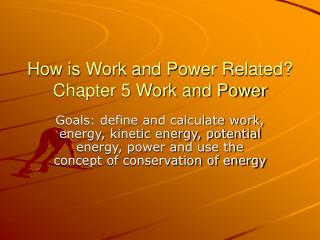# How is Work and Power Related? Chapter 5 Work and Power - PowerPoint PPT PresentationDownload PresentationHow is Work and Power Related? Chapter 5 Work and Power

How is Work and Power Related? Chapter 5 Work and PowerDownload Presentation## How is Work and Power Related? Chapter 5 Work and Power

- - - - - - - - - - - - - - - - - - - - - - - - - - - E N D - - - - - - - - - - - - - - - - - - - - - - - - - - -
##### Presentation Transcript

1. How is Work and Power Related? Chapter 5 Work and Power Goals: define and calculate work, energy, kinetic energy, potential energy, power and use the concept of conservation of energy

2. What is Work? Section 5.1 • Work is a transfer or conversion of energy • transfer occurs when a force is applied in the direction of the force force = mass x acceleration (g) • conversion occurs from one form of energy to another • mechanical, thermal, electrical, radiant, nuclear and chemical • W = F x d W = F x d cos θ

3. Simple Machines demonstrate the concept and calculations of Work • Simple Machine- device that makes work easier • multiply force OR distance • Increasing force or distance are at the expense of the other variable • Energy is conserved in an ideal situation no friction • Work in would equal Work out in another words F x d (in) = F x d (out) • Work in is done on the machine and Work out is done by the machine

4. R ---2m-----f--------4m------- E • MA = 2 • R= 40N---------f------------------- E = 20N • MA = 2 • MA = Fr / Fe or • MA = Xe / Xr • Levers include 1st, 2nd, 3rd, classes, wheels and axels and pulleys • Pulley- grooved wheel and a rope MA= the number of strands supporting resistance

5. Inclined Planes include ramps, wedges and screws • Gears- toothed wheels MA = ratio of teeth of each gear increase force or speed of rotation • Efficiency- ratio of work out to work in • eff = W out / W in

6. What is Energy? 5.2 • Energy – ability to cause a change • to do work work = transfer of energy • 6 forms of energy and two states of energy • Kinetic – motion • W = F x d • = m x a x ΔX a ΔX = vf² - vi² / 2 • = m x vf² - vi² / 2 • = ½ m v² • increase mass vs increase velocity v²

7. Work – Energy Theorem • transfer of energy by a force through a distance • transfers Kinetic Energy • Work = ΔKE • = KE f – KE i • Units KE = ½ mv² • = kg m²/s² • = N m • = J

8. What is Potential Energy? • Potential Energy – state of energy where a change is stored • position relative to a measured height • gravitational potential energy • PE = m g h weight = m g • = kg m/s² m = N • = N m • = J

9. Elastic potential energy – energy stored in an object stretched or compressed • Spring ex. spring scale • PE elastic = ½ k x² • k = spring constant • x = distance stretched

10. How is Energy Conserved ? • Conserved – energy is not gained or lost within a system • only converted or transferred • Mechanical Energy is the sum of the KE and the PE in a system • the exception is friction that converts some ME into heat that is nonmechanical • ME i = ME f KE + PE = KEtotal • ½ mv² + mgh = ½ mv² + mgh • ex. Falling rock PE to PE+KE to KE

11. What is the Rate at which work is done? • Power – the rate at which work is done or energy converted or transferred • P = ΔW / t • = F x d / t • = F x v • mgh / t or ½ mv² / t • units P = W / t • = J / s • = watts 1000 w = kw • 1 hp = 746 w

12. YOU have the POWER • To get the WORK done • And • Accelerate on this next Exam